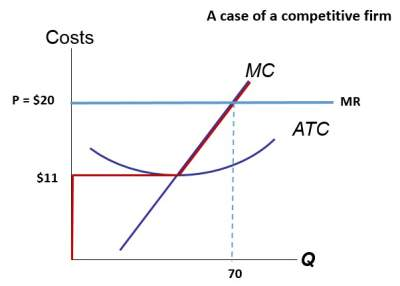# Exercise on Identifying a firm's profit: Solutions

Question: As illustrated in the figure, determine this firm's total profit.
Then calculate the firm's profit.Solution:
Profit per unit = P − ATC = \$20 − \$11 = \$19
Total profit = (P − ATC) × Q = \$19 × 70 = \$1,330